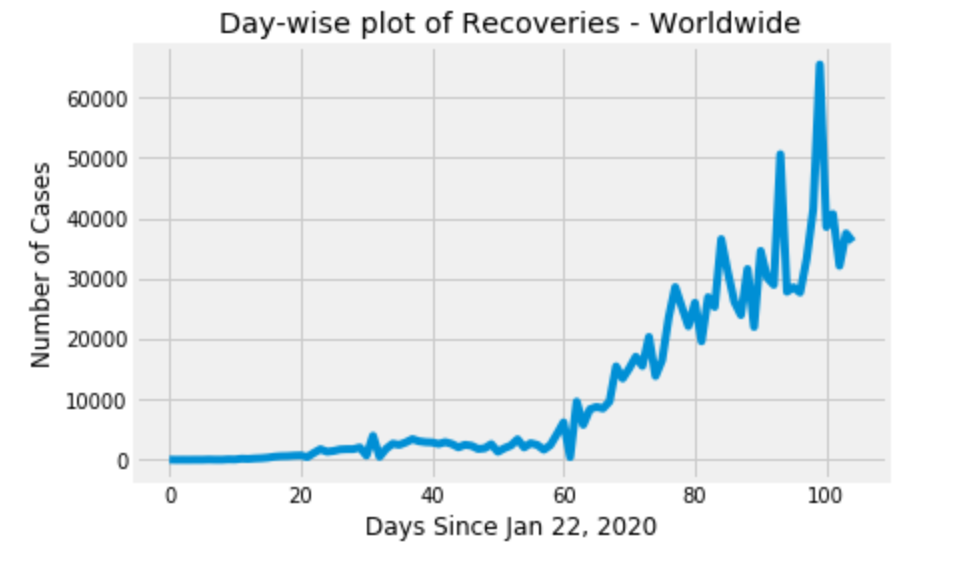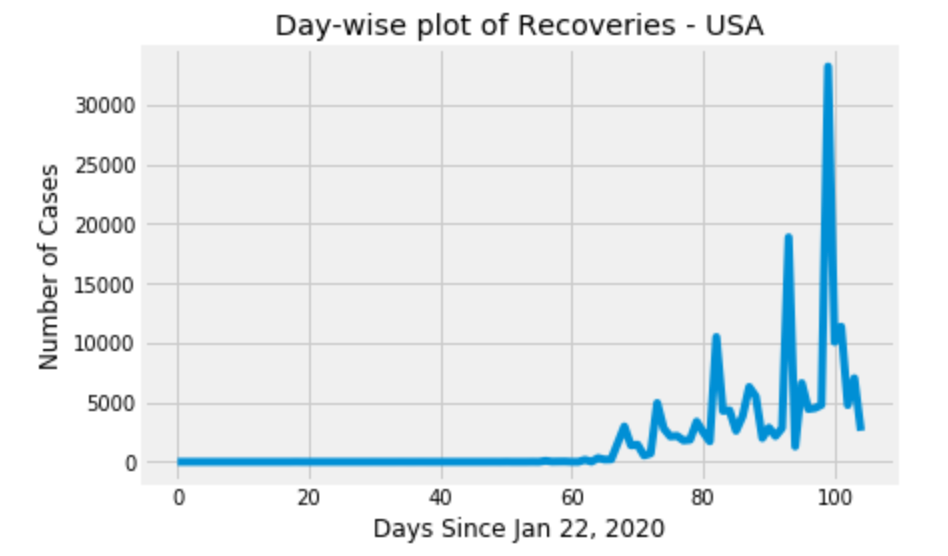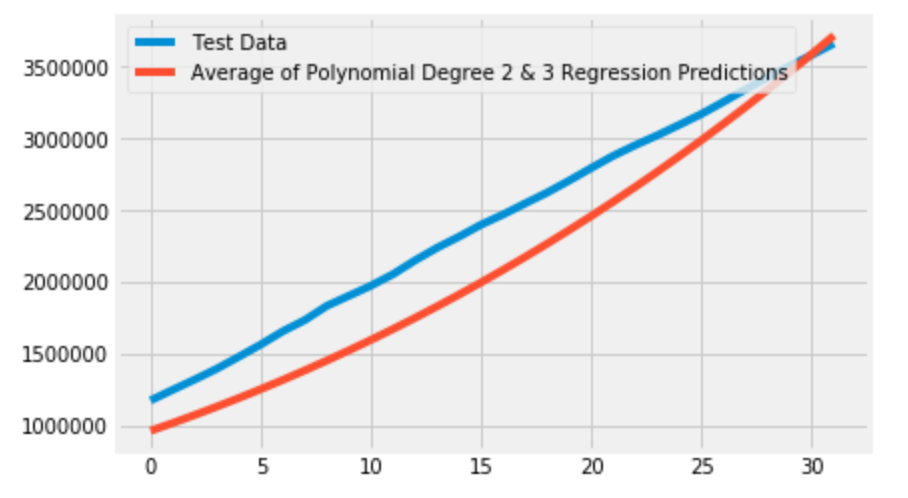# COVID-19 Data Analysis

## Introduction

The COVID-19 pandemic also known as coronavirus pandemic is the ongoing outbreak of coronavirus disease (COVID-19). It is caused by a coronavirus called severe acute respiratory syndrome coronavirus 2 (SARS‑CoV‑2).

The outbreak was identified in Wuhan, China, in December 2019. The World Health Organization declared the outbreak a Public Health Emergency of International Concern on 30 January, and a pandemic on 11 March.

It is a respiratory disease and is thought to spread mainly through close contact from person-to-person in respiratory droplets from someone who is infected. People who are infected often have symptoms of illness. Some people without symptoms may be able to spread virus. People may also become infected by touching a contaminated surface and then touching their face.

Common symptoms include fever, cough, fatigue, shortness of breath, and loss of smell. Complications may include pneumonia and acute respiratory distress syndrome. The time from exposure to onset of symptoms is typically around five days, but may range from two to fourteen days. There is no known vaccine or specific antiviral treatment. Primary treatment is symptomatic and supportive therapy.

This blog presents analysis, visualizations and predictions on COVID-19 pandemic data.

This analysis is divided into three parts:

1. Data Preparation
2. Visualization
3. Prediction

To follow along, please see the code that can be found in my GitHub profile.

## Data Preparation

As there are many data sources available online, the one used in this blog is provided by Johns Hopkins University Center for Systems Science and Engineering (JHU CSSE). This data is updated daily and for current visualizations, please run the notebook.

``````# import data from source

After loading the data, for visualizing and prediction purposes, data is sorted based on the columns and stored into lists.

``````# storing world data
world_cases = [] # to store total cases
total_deaths = [] # to store total deaths
mortality_rate = [] # to store mortality rate
recovery_rate = [] # to store recovery rate
total_recovered = []
total_active = []

# storing US data
us_cases = []
us_deaths = []
us_recoveries = []

for i in dates:
# calculate sums
confirmed_sum = confirmed[i].sum()
death_sum = deaths[i].sum()
recovered_sum = recoveries[i].sum()

# confirmed, deaths, recovered, and active
world_cases.append(confirmed_sum)
total_deaths.append(death_sum)
total_recovered.append(recovered_sum)
total_active.append(confirmed_sum-death_sum-recovered_sum)

# calculate rates
mortality_rate.append(death_sum/confirmed_sum)
recovery_rate.append(recovered_sum/confirmed_sum)

# case studies
us_cases.append(confirmed_df[confirmed_df['Country/Region']=='US'][i].sum())
us_deaths.append(deaths_df[deaths_df['Country/Region']=='US'][i].sum())
us_recoveries.append(recoveries_df[recoveries_df['Country/Region']=='US'][i].sum())

def get_daily_increase(data):
'''
INPUT - a list containing day by day case counts

OUTPUT - a list containing the day by day increment of count

Function to count the daily increment in figures
'''
increment_count = []
for i in range(len(data)):
if i == 0:
increment_count.append(data)
else:
increment_count.append(data[i]-data[i-1])
return increment_count

# confirmed cases
world_daily_increase = get_daily_increase(world_cases)
us_daily_increase = get_daily_increase(us_cases)

# deaths
world_daily_death = get_daily_increase(total_deaths)
us_daily_death = get_daily_increase(us_deaths)

# recoveries
world_daily_recovery = get_daily_increase(total_recovered)
us_daily_recovery = get_daily_increase(us_recoveries)

# days from the first day in the dataset i.e. Jan 22, 2020 (1/22/2020)
days = np.array([i for i in range(len(dates))]).reshape(-1, 1)

# reshaping the data
world_cases = np.array(world_cases).reshape(-1, 1)
total_deaths = np.array(total_deaths).reshape(-1, 1)
total_recovered = np.array(total_recovered).reshape(-1, 1)``````

Storing Data into various data structures for visualization and prediction

Of the different lists, one is for storing mortality rate and another is for storing recovery rate.

Mortality rate can be defined as the ratio of number of deaths recorded against the total number of cases recorded and this is calculated using the following formula:

Recovery rate can be defined as the ratio of number of recovered patients recorded against the total number of cases recorded and this is calculated using the following formula:

Now, as the data is sorted into various lists and stored, it is time for visualizations.

## Visualization

As the data is loaded, prepared, and stored; the worldwide stats are plotted first. The data used here was recorded from January 22, 2020 (and is being updated on a daily basis).

### Worldwide statistics

Let’s start with a couple of graphs showing the overall situation of the total cases.

The images are related to data updated on 5th May, 2020.

This is how a pandemic looks like, with a huge growth of the positive cases and the relative outcomes, with count of deaths fortunately less than that of the count of recoveries.

Now, let’s see a breakdown of the day-wise counts.Day wise plots (a) confirmed cases (b) deaths (c ) recoveries — Worldwide

This shows how random the day wise growth of the cases and relative outcomes is. Let’s look at changes in mortality rate and recovery rate of the deadly COVID-19 pandemic.

Now, let’s plot the US pandemic data.Day wise plots (a) confirmed cases (b) deaths (c ) recoveries — USA wide

As we have have the plots for day wise counts in USA, let’s see the top-10 states/regions with the most confirmed cases. The remaining states are grouped into “others” category

Now, we move onto predicting the future cases.

## Predictions

In this section, we’ll be predicting the rise in cases for the next fifteen days using variants of Linear Regression algorithm of Python’s scikit-learn library.

The first one we use is a basic Linear Regression model.

From the plot it can be seen that basic Linear Regression model’s predictions are no where near the test data.

The next variant we’ll try is the Polynomial Regression. That is the we convert the features into polynomial features.

For this we use scikit-learn’s PolynomialFeatures class. This class generates a new feature matrix consisting of all polynomial combinations of the features with degree less than or equal to the specified degree. For example, if an input sample is two dimensional and of the form [a, b], the degree-2 polynomial features are [1, a, b, a², ab, b²]

It can be seen that of the three models, Polynomial Regression of Degree-3 performed well on the test data. But, this model’s future predictions are very large numbers. Hence, we average predictions of Polynomial Regression of Degree-2 &3 models.Average of Polynomial Regression models of Degree — 2 & 3

The future predictions by the three algorithms and the average of polynomial models can be seen in the graph below.

Note: This is just a simple model and the results are not accurate. For more accurate predictions, try using other regression or deep learning techniques.

## Summary

In this article, we discussed about COVID-19 pandemic and have done some analysis on the data provided by Johns Hopkins University.

We also have seen some visualizations of data that is currently available, and also tried to predict the number of cases that are likely to occur in future.

These are just simple examples of possible reports that can help to comprehend more easily the magnitude of what is happening.

There are many factors that can change these predictions. How the data is collected could affect the predictions and in this dataset, currently, there are missing features that could help, like statistics about age intervals of positives, recovers and deaths.